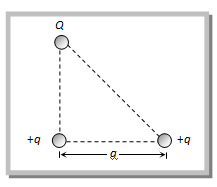# Three charges Q, +q and +q are placed at the vertices of a right-angled isosceles triangle as shown. The net electrostatic energy of the configuration is zero if Q is equal toOption 1) Option 2) Option 3) -2q Option 4) +q

V Vakul

As we have learnt,

Potential Energy Of System Of two Charge -

- wherein

Potential energy of the configuration is

Option 1)

Option 2)

Option 3)

-2q

Option 4)

+q

Exams
Articles
Questions